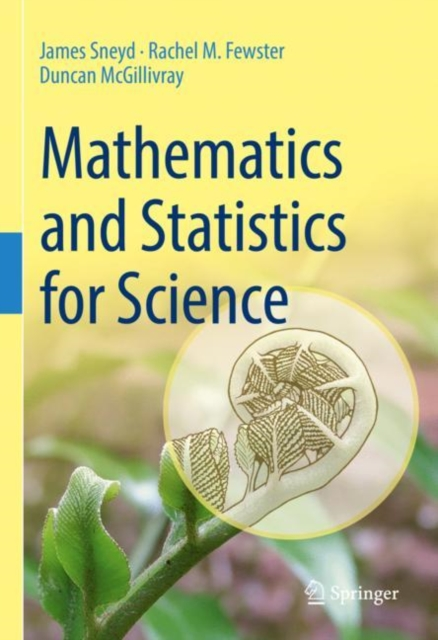# Mathematics and Statistics for Science Hardback

Hardback

• Information

#### Description

Mathematics and statistics are the bedrock of modern science.

No matter which branch of science you plan to work in, you simply cannot avoid quantitative approaches. And while you won't always need to know a great deal of theory, you will need to know how to apply mathematical and statistical methods in realistic scenarios.

That is precisely what this book teaches. It covers the mathematical and statistical topics that are ubiquitous in early undergraduate courses, but does so in a way that is directly linked to science. Beginning with the use of units and functions, this book covers key topics such as complex numbers, vectors and matrices, differentiation (both single and multivariable), integration, elementary differential equations, probability, random variables, inference and linear regression.

Each topic is illustrated with widely-used scientific equations (such as the ideal gas law or the Nernst equation) and real scientific data, often taken directly from recent scientific papers.

The emphasis throughout is on practical solutions, including the use of computational tools (such as Wolfram Alpha or R), not theoretical development.

There is a large number of exercises, divided into mathematical drills and scientific applications, and full solutions to all the exercises are available to instructors. Mathematics and Statistics for Science covers the core methods in mathematics and statistics necessary for a university degree in science, highlighting practical solutions and scientific applications.

Its pragmatic approach is ideal for students who need to apply mathematics and statistics in a real scientific setting, whether in the physical sciences, life sciences or medicine.

£79.99

£56.80

£79.50

£67.58

£66.00

£46.11

£109.99

£76.27

£72.00

£61.20